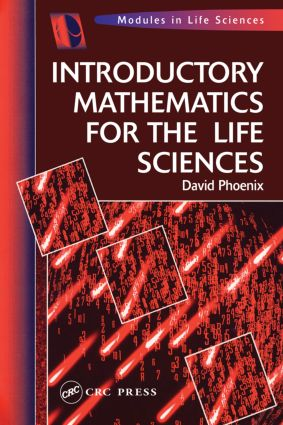# Introductory Mathematics for the Life Sciences

## 1st Edition

CRC Press

229 pages

Paperback: 9780748404285
pub: 1997-09-02
Currently out of stock
\$52.95
x
Hardback: 9781138442054
pub: 2018-01-30
\$215.00
x
eBook (VitalSource) : 9781315272733
pub: 2018-10-24
from \$26.48

FREE Standard Shipping!

### Description

Introductory Mathematics for the Life Sciences offers a straightforward introduction to the mathematical principles needed for studies in the life sciences. Starting with the basics of numbers, fractions, ratios, and percentages, the author explains progressively more sophisticated concepts, from algebra, measurement, and scientific notation through the linear, power, exponential, and logarithmic functions to introductory statistics. Worked examples illustrate concepts, applications, and interpretations, and exercises at the end of each chapter help readers apply and practice the skills they develop. Answers to the exercises are posted at the end of the text.

Numbers. Fractions, percentages and ratios. Basic algebra & measurement. Powers and scientific notation. Concentration and accuracy. Tables, charts and graphs. Linear functions. Power functions. Exponential functions. Logarithmic Functions. Introduction to statistics. Appendix: Solution to problems.